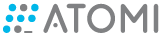# Run a script that changes an objects state on variable change

Problem:
I’m having issues with my current JavaScript (Still new to the idea of scripts with ActivePresenter.) I’m attempting to have an object change states when a button is clicked.

I’ve assigned a variable to increase by 1 every time the button is clicked, and then simultaneously run the following script:

//When button is pressed, the variable change is increased by 1
//The following variables represent the three cases
var casA = prez.variable(‘Change’) == 1;
var casB = prez.variable(‘Change’) == 2;
var casC = prez.variable(‘Change’) == 3;

//represents the object square
var square = prez.object(“Square”)

//this section is supposed to change the state of the object, but doesn’t work when running the presentation.
if (casA)
{

``````prez.object("Square").state(State2)
``````

}

else if (casB)
{

``````prez.object("Square").state(State3)
``````

}

else if (casC)
{

``````prez.object("Square").state(State4)
``````

}

I’d love to hear any input, thank you

ActivePresenter version: 8

OS: Wubdiws

Notes:

Hi,

A state name is a literal string so that it must be single quoted (`'`) or double quoted (`"`).
You can use the script below to update your object state

``````// state names
var states = ['Normal', 'State2', 'State3', 'State4'];
// increase Change variable by 1
var change = prez.variable('Change');
++change;
prez.variable('Change', change);
// change object state
if (change >= 0 && change < states.length)
prez.object('Square').state(states[change]);
``````

Regards

Oh I See!!

Thank you so much, this helps a lot